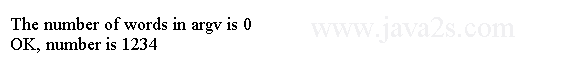# Java Tutorial - Java Method

Classes usually consist of two things: instance variables and methods. Instance variables are the data part of a class, while the methods defines the behaviours of a class.

## Syntax

This is the general form of a method:

```type name(parameter-list) {
// body of method
}
```

type specifies the type of data returned by the method. If the method does not return a value, its return type must be void. The name of the method is specified by name.

The parameter-list is a sequence of type and identifier pairs separated by commas.

Parameters receives the value of the arguments passed to the method.

If the method has no parameters, then the parameter list will be empty.

Add a method to Box,as shown here:

```
class Box {/*from   w w w  .  j  a v  a 2s . c o  m*/
int width;
int height;
int depth;
void calculateVolume() {
System.out.print("Volume is ");
System.out.println(width * height * depth);
}
}

public class Main {

public static void main(String args[]) {
Box mybox1 = new Box();
mybox1.width = 10;
mybox1.height = 20;
mybox1.depth = 15;

mybox1.calculateVolume();

}
}
```

This program generates the following output: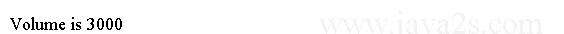## Java Method Return

A method in a class can return a value with the return statement.

Methods that have a return type other than void return a value to the calling routine using the following form of the return statement:

```return value;
```

Here, value is the value returned.

We can use `return` statement to return a value to the callers.

```
class Rectangle {
int width;//from w  ww .jav  a  2s.  c o  m
int height;

int getArea() {
return width * height;
}
}

public class Main {

public static void main(String args[]) {
Rectangle mybox1 = new Rectangle();
int area;
mybox1.width = 10;
mybox1.height = 20;

area = mybox1.getArea();
System.out.println("Area is " + area);

}
}
```

The output: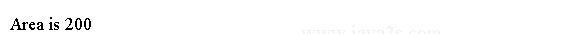In this line the return statement returns value from the `getArea()`method. And the returned value is assigned to `area`.

```area = mybox1.getArea();
```

The actual returned data type must be compatible with the declared return type . The variable receiving the returned value (`area`) must be compatible with the return type.

The following code uses the returned value directly in a println( ) statement:

```System.out.println("Area is " + mybox1.getArea());
```

## Example

A method can return class types.

```
class MyClass {/*from  w ww  .ja  va  2s .  c o  m*/
int myMemberValue = 2;
MyClass() {
}
MyClass doubleValue() {
MyClass temp = new MyClass();
temp.myMemberValue = temp.myMemberValue*2;
return temp;
}
}

public class Main {
public static void main(String args[]) {
MyClass ob1 = new MyClass();
ob1.myMemberValue =2;
MyClass ob2;

ob2 = ob1.doubleValue();
System.out.println("ob1.a: " + ob1.myMemberValue);
System.out.println("ob2.a: " + ob2.myMemberValue);

ob2 = ob2.doubleValue();
System.out.println("ob2.a after second increase: " + ob2.myMemberValue);
}
}
```

The output generated by this program is shown here: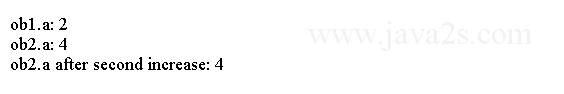## Java Method Parameters

Parameters allow a method to be generalized by operating on a variety of data and/or be used in a number of slightly different situations.

This is the general form of a method:

```type methodName(parameterType variable,parameterType2 variable2,...) {
// body of method
}
```

A parameterized method can operate on a variety of data.

The new `Rectangle` class has a new method which accepts the dimensions of a rectangle and sets the dimensions with the passed-in value.

```
class Rectangle {
double width;/* w  w  w  .jav a  2 s .c  om*/
double height;

double area() {
return width * height;
}

void setDim(double w, double h) { // Method with parameters
width = w;
height = h;
}
}

public class Main {

public static void main(String args[]) {
Rectangle mybox1 = new Rectangle();
double vol;
mybox1.setDim(10, 20);

vol = mybox1.area();
System.out.println("Area is " + vol);

}
}
```

The output: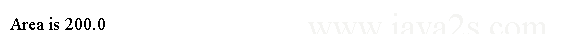## Example 2

The following code passes objects to methods.

```
class Test {/*  w w  w. ja v a 2  s.  co m*/
int a;

Test(int i) {
a = i;
}
boolean equals(Test o) {
if (o.a == a )
return true;
else
return false;
}
}

public class Main {
public static void main(String args[]) {
Test ob1 = new Test(100);
Test ob2 = new Test(100);

System.out.println("ob1 == ob2: " + ob1.equals(ob2));

}
}
```

This program generates the following output: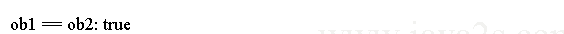Java allows to define two or more methods within the same class that share the same name, as long as their parameter declarations are different.

When this is the case, the methods are said to be overloaded, and the process is referred to as method overloading.

Overloaded methods have the same name but different parameters. Overloaded methods must differ in the type and/or number of their parameters. Overloaded methods may have different `return` types. `return` type alone is insufficient to distinguish two methods.

```
void test() {// w  ww  .ja v  a  2  s.c o  m
System.out.println("No parameters");
}
void test(int a) {
System.out.println("a: " + a);
}
void test(int a, int b) {
System.out.println("a and b: " + a + " " + b);
}
double test(double a) {
System.out.println("double a: " + a);
return a * a;
}
}
public class Main {
public static void main(String args[]) {
ob.test();
ob.test(10);
ob.test(10, 20);
double result = ob.test(123.25);
System.out.println("Result of ob.test(123.25): " + result);

}
}
```

This program generates the following output: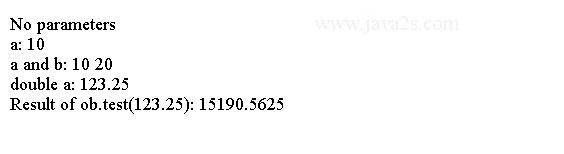## Example 3

```
void test() {//from ww  w.jav  a  2 s  .c  o  m
System.out.println("No parameters");
}
void test(int a, int b) {
System.out.println("a and b: " + a + " " + b);
}
void test(double a) {
System.out.println("Inside test(double) a: " + a);
}
}

public class Main {
public static void main(String args[]) {
int i = 88;
ob.test();
ob.test(10, 20);

ob.test(i); // this will invoke test(double)
ob.test(123.2); // this will invoke test(double)
}
}
```

This program generates the following output: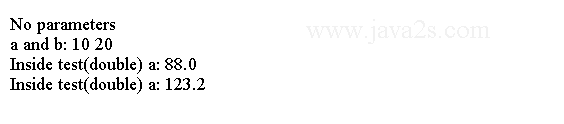## Java Method Recursion

Recursion allows a method to call itself.

The following code is an example of recursion. It calculates the factorial numbers.

```
class Factorial {
// this is a recursive function
int fact(int n) {
int result;//w  w  w  .  j a  v a 2s . c  o m

if (n == 1)
return 1;
result = fact(n - 1) * n;
return result;
}
}

public class Main {
public static void main(String args[]) {
Factorial f = new Factorial();

System.out.println("Factorial of 5 is " + f.fact(5));

}
}
```

The output from this program is shown here: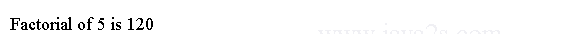## Java main() Method

The `main()` method is the entry point for standalone Java applications. To create an application, you write a class definition that includes a `main()` method. To execute an application, type java at the command line, followed by the name of the class containing the `main()` method.

## Syntax for main() method

The signature for main() is:

```public static void main(String[] args)
```

The return type must be `void`. The main() method must be `public`. It is static so that it can be executed without constructing an instance of the application class.

A command-line argument is the information that follows the program's name on the command line. The command-line arguments are stored as string array passed to main(). For example, the following program displays all of the command-line arguments:

```
public class Main {
public static void main(String args[]) {
for (int i = 0; i < args.length; i++)
System.out.println("args[" + i + "]: " + args[i]);
}
}
```

Try executing this program, as shown here: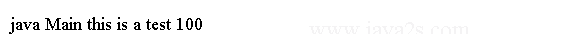When you do, you will see the following output: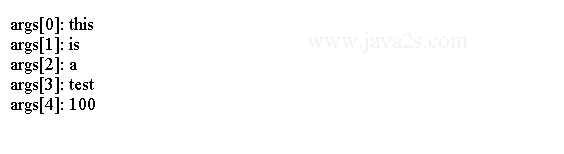## Example 4

The following code shows how to use of argv to get an integer value from command line.

```// ww  w  . java2 s  .c  o m
public class Main {
public static void main(String[] argv) {
int number = 0;

System.out.println("The number of words in argv is " + argv.length);

if (argv.length == 0) {
number = 1234;
} else if (argv.length == 1) {
try {
number = Integer.parseInt(argv);
} catch(NumberFormatException e) {
System.err.println("Number " + argv + " invalid (" + e.getMessage() + ").");
System.exit(1);
}
} else {
System.err.println("usage: UseArgv number");
System.exit(1);
}

System.out.println("OK, number is " + number);
}
}
```

The code above generates the following result.